# AP Calculus BC Question 63: Answer and Explanation

### Test Information

Question: 63

8. The sum of the infinite geometric series 2 -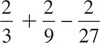+…is

• A. -6
• B. -3
• C.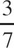• D.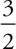D This is the geometric series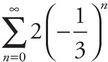. The sum of an infinite series of the form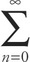arn is S =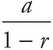. Here, the sum is S =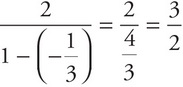.